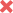# Wave's fundamental frequency

Any Help

## Homework Statement

A vibrating tuning fork of frequency 512 Hz is held over a water column with one end closed and the other open. As the water level is allowed to fall, a loud sound (resonance) is heard at specific water levels. Assume you start with the tube full of water, and begin steadily lowering the water level. What is the water level (as measured from the top of the tube) for the third such resonance? Take the speed of sound in air to be 343 m/s.

• A

83.7 cm

• B

16.7 cm

• C

33.5 cm

•D

50.2 cm

• E

167 cm

## Homework Equations

in a fundamental frequency (f):
λ=2L/n and f=n/2L .v for waves whose both ends are open
λ=4L/n and f=n/4L .v for waves whose one end closed and the second is open
n is a positive integer; n= 0,1,2,3,4,5,6....
v is the velocity
L is the length of string
λ is the wavelength

## The Attempt at a Solution

here we have one open and other closed ends then we use λ=4L/n and f=n/4L .v
so f=512Hz, n=3 (third such resonance) and v=343m/sec
substitute in the equation
we get L equal 0.502m=50.2cm then it is D
but why the correct answer is A??

Last edited by a moderator:

## Answers and Replies

Homework Helper
Gold Member
n = 3 is incorrect. The best thing to do for these types of problems is to draw a diagram of the standing wave for the situation. You can then determine L from the diagram.

Last edited:
Any Help
n = 3 is incorrect. The best thing to for these types of problems is to draw a diagram of the standing wave for the situation. You can then determine L from the diagram.
I didn't get your point. How can I draw diagram for it? Besides why n=3 is not correct? what do they mean by the third such resonance?

Homework Helper
Gold Member
How can I draw diagram for it?
When the level of the water is at a position of resonance, what can you say about the positions of nodes and antinodes, especially at the top of the tube and at the top of the water? You can use this knowledge as a guide for drawing the various cases where you get resonance.
Besides why n=3 is not correct?
The n in the formula does not necessarily correspond to the number of the resonance. Thus n = 3 does not correspond to the third resonance in your situation.
What do they mean by the third such resonance?
Imagine the water level starts at the top of the tube. As the level of the water is lowered in the tube while the fork is vibrating, there will be certain positions of the level of the water that will cause the sound from the fork to resonate. The first level that produces a resonance is the first resonance. The next level of water that produces resonance is the second resonance. And so on.

Last edited: